# JP4167811B2 - Switching power supply - Google Patents

## Info

Publication number
JP4167811B2
JP4167811B2 JP2001059467A JP2001059467A JP4167811B2 JP 4167811 B2 JP4167811 B2 JP 4167811B2 JP 2001059467 A JP2001059467 A JP 2001059467A JP 2001059467 A JP2001059467 A JP 2001059467A JP 4167811 B2 JP4167811 B2 JP 4167811B2
Authority
JP
Japan
Prior art keywords
circuit
output
value
inductor
inductor current
Prior art date
Legal status (The legal status is an assumption and is not a legal conclusion. Google has not performed a legal analysis and makes no representation as to the accuracy of the status listed.)
Active
Application number
JP2001059467A
Other languages
Japanese (ja)
Other versions
JP2002262565A (en
Inventor

Original Assignee
Ｔｄｋ株式会社
Priority date (The priority date is an assumption and is not a legal conclusion. Google has not performed a legal analysis and makes no representation as to the accuracy of the date listed.)
Filing date
Publication date
Application filed by Ｔｄｋ株式会社 filed Critical Ｔｄｋ株式会社
Priority to JP2001059467A priority Critical patent/JP4167811B2/en
Publication of JP2002262565A publication Critical patent/JP2002262565A/en
Application granted granted Critical
Publication of JP4167811B2 publication Critical patent/JP4167811B2/en
Application status is Active legal-status Critical
Anticipated expiration legal-status Critical

## Images

••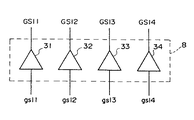•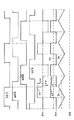•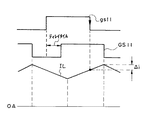•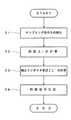••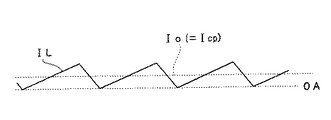•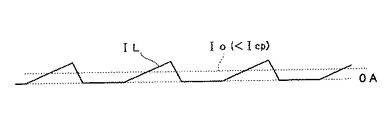••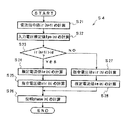•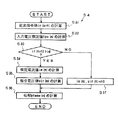•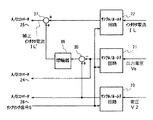•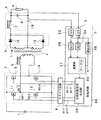•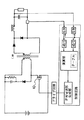## Description


BACKGROUND OF THE INVENTION
The present invention relates to a switching power supply device, and more particularly to a switching power supply device capable of obtaining correct operation timing.

[Prior art]
Conventionally, what is called a DC / DC converter is known as a switching power supply device. A typical DC / DC converter converts a DC input to AC once using a switching circuit, then transforms it (steps up or down) using a transformer, and further converts it to DC using an output circuit. Thus, a DC output having a voltage different from the input voltage can be obtained.

Here, a choke input type smoothing circuit may be used as an output circuit used in the DC / DC converter. When a choke input type smoothing circuit is used as the output circuit of the DC / DC converter, the operation of the switching circuit is controlled so that the output voltage becomes a constant value by monitoring the output voltage, the inductor current, and the like.

As an example of performing such operation control, there is a switching power supply device described in JP-A-11-206119.

In the switching power supply device described in the publication, the operation timing is determined by sampling the output voltage, the inductor current, and the like at a predetermined timing and performing a calculation according to a predetermined algorithm based on the sampling.

[Problems to be solved by the invention]
In general, since a switching element (transistor or the like) having a large driving capability is used in a switching circuit of a switching power supply device, a drive circuit is often used to control on / off of the switching element. On the other hand, the data such as the inductor current used for the calculation needs to use a value at a predetermined timing, for example, a timing when the switch element is turned off.

However, since the drive circuit has a predetermined delay, a predetermined shift occurs between the timing when switching is instructed and the timing when the switch element is actually turned off. For this reason, when the inductor current or the like is sampled in response to the timing for instructing switching, the sampling timing deviates from a desired timing, and data including an error is sampled. Due to such an error, the operation timing obtained as a result of the calculation is also distorted, so that the correct operation timing of the switching circuit cannot always be obtained.

Therefore, an object of the present invention is to provide a switching power supply device that can obtain correct operation timing even when a delay exists in a signal path for controlling a switching element of a switching circuit.

[Means for Solving the Problems]
Such an object of the present invention is to provide a switching circuit connected to a DC input power source, a first inductor connected to one output of the switching circuit, and a connection between the other output of the switching circuit and the inductor. A transformer having a primary winding formed; Transformer An output rectifier circuit that rectifies the output voltage of the transformer and is connected to the output rectifier circuit and includes at least a second inductor, and smoothes the output voltage of the output rectifier circuit. A current detection circuit configured to detect a value of an inductor current flowing in the second inductor in response to an instruction of a switching operation to the switching circuit, a control circuit; a drive circuit that controls on / off of the switching circuit; And the control circuit is detected by the current detection circuit. Above Correction means for correcting the value IL of the inductor current, the correction means based on the following equation (1):
Δi = (V2−V0) Tdelay / Lf (Formula 1)
An error Δi between the inductor current value IL detected by the current detection circuit and the inductor current peak value IL ′ is calculated (where V2 is the output voltage of the output rectifier circuit, and V0 is the output smoothing). The output voltage of the circuit, and Tdelay is the delay time of the output signal to the switching circuit of the drive circuit with respect to the input signal input from the control circuit to the drive circuit.), Based on the following equation (2) Then, the switching power supply device drives the drive circuit by substantially correcting the inductor current value IL detected by the current detection circuit to a peak value IL ′ of the inductor current.
IL ′ = IL + Δi (Formula 2)

According to the present invention, Drive circuit for controlling on / off of switching circuit Even if there is a delay in The value IL of the inductor current detected by the current detection circuit is substantially corrected to the peak value IL ′ of the inductor current. From Drive circuit It is possible to obtain a correct inductor current value in consideration of the delay, and therefore it is possible to obtain a correct operation timing.

In a preferred embodiment of the present invention, the correction of the value IL of the inductor current detected by the current detection circuit by the correction means is executed by software.

Of the present invention another In a preferred embodiment, Calculation of the error Δi by the correction means and correction of the inductor current value IL detected by the current detection circuit At least one of these is executed in hardware.

The object of the present invention is also a DC-AC converting means for converting a DC input into an AC by a switching operation, an AC-DC converting means having at least an inductor and converting the AC into a DC output, and the DC-AC conversion. A control circuit for controlling the switching operation of the means, wherein the control circuit periodically detects the value of the inductor current flowing through the inductor, and at least based on a detection result by the first means. And a second means for instructing the DC-AC conversion means to perform the switching operation, and the detection by the first means has been performed for a predetermined time after the instruction is given by the second means. This is achieved by a switching power supply characterized by being performed later.

According to the present invention, even when there is a delay in the signal path for controlling the switching operation by the DC / AC converting means, the detection of the inductor current is performed based on the instruction of the switching operation to the DC / AC converting means. Since the process is performed after a lapse of time, a correct inductor current value can be obtained in consideration of the delay. For this reason, it is possible to obtain correct operation timing.

In a preferred embodiment of the present invention, after the predetermined time, the second means instructs the DC-AC conversion means to perform the switching operation, and then the DC-AC conversion means performs the switching operation. Is substantially equal to the delay time until.

DETAILED DESCRIPTION OF THE INVENTION
Hereinafter, preferred embodiments of the present invention will be described in detail with reference to the accompanying drawings.

FIG. 1 is a circuit diagram showing a switching power supply device according to a preferred embodiment of the present invention.

As shown in FIG. 1, the switching power supply according to this embodiment includes a switching circuit 2 connected to a DC input power supply 1, an inductor 3 connected to one output of the switching circuit 2, and a switching circuit 2. The main transformer 4 having a primary winding connected between the other output and the inductor 3, the output rectifier circuit 5 connected to the secondary winding of the main transformer 4, and the output rectifier circuit 5 An output smoothing circuit 6, a control circuit 7, and a drive circuit 8 are provided, and the output of the output smoothing circuit 6 is connected to a load 9.

The switching circuit 2 includes switch elements 11 and 12 connected in series between the DC input power supplies 1 and switch elements 13 and 14 connected in series between the DC input power supplies 1. The node is connected to one end of the primary winding of the main transformer 4 via the inductor 3, and the node of the switch elements 13 and 14 is directly connected to the other end of the primary winding of the main transformer 4. As shown in FIG. 1, in this embodiment, n-channel MOSFETs are used as the switch elements 11 to 14.

The inductor 3 forms a resonance circuit together with the parasitic capacitance of the switch elements 11 to 14. As a result, the switching elements 11 to 14 can be turned on by ZVS (Zero Voltage Switching), so that the switching loss is reduced and the generation of noise is suppressed.

The main transformer 4 is a transformer in which the turns ratio of the primary winding and the secondary winding is 1: m, and includes two secondary windings as shown in FIG. The midpoint of these two secondary windings is connected to the negative terminal of the load 9. Hereinafter, the voltage generated in the primary winding of the main transformer 4 is defined as V1.

The output rectifier circuit 5 includes two diodes 15 and 16 each having an anode connected to the end of the secondary winding of the main transformer 4, and the cathodes of these two diodes are connected in common. Hereinafter, the common cathode connection point of these two diodes 15 and 16 is referred to as “rectification point”, and the voltage appearing here is defined as V2.

The output smoothing circuit 6 includes an inductor 17 connected between the rectification point and the positive terminal of the load 9, and a capacitor 18 connected between both ends of the load 9. Hereinafter, the voltage appearing across the load 9 is referred to as “output voltage (Vo)”, and the current flowing through the load 9 is referred to as “output current (Io)”. The current flowing through the inductor 17 is referred to as “inductor current IL”. As shown in FIG. 1, a current detection circuit 19 that detects the inductor current IL is provided between the negative terminal of the load 9 and the main transformer 4.

The control circuit 7 samples a sample / hold circuit 21 that samples / holds the output voltage Vo, a sample / hold circuit 22 that samples / holds the inductor current IL detected by the current detection circuit 19, and samples the voltage V2 at the rectification point. A sample / hold circuit 23 to be held / held, A / D converters 24 to 26 for converting analog values held in the sample / hold circuits 21 to 23 to digital values, and from the A / D converters 24 to 26, respectively. A calculation unit 27 that performs a predetermined calculation based on the digital output, and a phase control signal generation unit 28 that generates phase control signals (gs11 to gs14) to be supplied to the switch elements 11 to 14 based on the calculation result of the calculation unit 27; Is provided. Sampling by the sample / hold circuits 21 and 22 is performed in response to the sampling signal S generated by the arithmetic unit 27.

FIG. 2 is a circuit diagram schematically showing the internal configuration of the drive circuit 8.

As shown in FIG. 2, the drive circuit 8 includes four drivers 31 to 34. The drivers 31 to 34 receive the corresponding phase control signals (gs11 to gs14) and amplify them to voltage and current levels that can drive the switch elements 11 to 14 of the switching circuit 2 (GS11 to GS14). Are supplied to the gates of the corresponding switch elements 11 to 14, respectively. Since the drivers 31 to 34 all have a predetermined delay, the waveform of the output phase control signals (GS11 to GS14) is more relevant than the waveform of the input phase control signals (gs11 to gs14). The waveform is delayed by the delay amount.

FIG. 3 is an operation waveform diagram showing the basic operation of the switching power supply device according to this embodiment.

The basic operation of the switching power supply according to this embodiment is as follows. That is, the input voltage Vin from the DC input power source 1 is applied to the primary winding of the main transformer 4 in one direction during a period when both GS11 and GS14 are at a high level, that is, during a period when both the switch elements 11 and 14 are on. The input voltage Vin from the DC input power source 1 is applied in the reverse direction to the primary winding of the main transformer 4 during a period in which both GS12 and GS13 are at a high level, that is, both the switch elements 12 and 13 are on. The voltage V1 generated in the primary winding of the main transformer 4 is changed to an AC waveform. The peak value of the AC waveform is multiplied by m in the secondary winding of the main transformer 4 and further rectified by the output rectifier circuit 5.

Here, in the period in which both GS11 and GS14 are high in FIG. 3 and in the initial period in which GS12 and GS13 are both high, the voltages V1 and V2 are not generated, and the inductor current IL is The reason why it continues to fall is because the energy regeneration of the inductor 17 has not ended.

As shown in FIG. 3, the waveform of the voltage V2 at the rectification point is a pulse waveform having a predetermined width whose peak value is Vin × m, and the time average value thereof is the output voltage Vo. Therefore, in order to control the value of the output voltage Vo, the phases of GS11 and GS14 and the phases of GS12 and GS13 may be controlled. Further, the waveform of the inductor current IL is a waveform in which a ripple current determined by the voltage waveform V2 at the rectification point and the inductance of the inductor 17 is superimposed on the output current Io.

In order to maintain the output voltage Vo at a desired constant value, it is necessary to control the phase difference between the phase control signals (gs11 and gs14) and the phase difference between the phase control signals (gs12 and gs13). Is performed by performing a predetermined calculation based on the result of sampling performed every integer multiple of one period of the phase control signal generated by the phase control signal generation unit 28. In order to perform such calculation correctly, as an example, it is necessary to perform sampling at the peak of the inductor current IL.

Here, in order to perform sampling at the peak of the inductor current IL, the sampling signal S may be generated in response to the fall of the phase control signal GS11 or GS12. As described above, the drive circuit 8 is configured. Due to the delay of the drivers 31 to 34, the waveforms of the phase control signals (GS11 to GS14) output to the drive circuit 8 and the waveforms of the input phase control signals (gs11 to gs14) do not match. On the other hand, the various internal signals in the calculation unit 27 substantially match the waveforms of the phase control signals (gs11 to gs14). Therefore, when the sampling signal S is generated using such internal signals, the sampling signal S The timing deviates from the peak of the inductor current IL.

FIG. 4 is an enlarged timing diagram showing the relationship between the phase control signals (gs11 to gs14) and the phase control signals (GS11 to GS14) and the waveform of the inductor current IL.

As shown in FIG. 4, if the value of the inductor current IL is sampled in response to the falling edge of the phase control signal gs11, the obtained value is a value smaller than the peak of the inductor current IL by an error Δi. It becomes. The same applies to the case where the value of the inductor current IL is sampled in response to the falling edge of the phase control signal gs12.

For this reason, in the switching power supply according to this embodiment, the inductor current IL is corrected in consideration of the error Δi. Hereinafter, a method for correcting the inductor current IL and a method for determining the phase of the phase control signals (gs11 and gs14) and the phase of the phase control signals (gs12 and gs13) based on the corrected inductor current IL (corrected inductor current IL ′). This will be described in detail.

FIG. 5 is a flowchart showing an algorithm for determining the operation timing of the switching circuit 2. Calculations according to such an algorithm are executed in software in the calculation unit 27.

First, when the arithmetic unit 27 generates the sampling signal S in response to the generation timing of the phase control signal gs11, the sampling by the sample / hold circuits 21 to 23 is performed in response to this (step S1). As described above, the sample / hold circuit 21 samples the output voltage Vo, the sample / hold circuit 22 samples the inductor current IL detected by the current detection circuit 19, and the sample / hold circuit 23 uses the rectification point voltage V2. Sampling. The output voltage Vo, the inductor current IL, and the voltage V2, which are analog values held in the sample / hold circuits 21 to 23, are converted into digital signals by the A / D converters 24 to 26, respectively, and supplied to the arithmetic unit 27. The

Upon receiving such a digital signal, the calculation unit 27 first calculates an error Δi based on the equation (1) (step S2).

[Expression 1]
Here, Tdelay is a delay time of the phase control signals (GS11 to GS14) with respect to the phase control signals (gs11 to gs14), and substantially coincides with the delay times of the drivers 31 to 34 constituting the drive circuit 8. However, if there are factors that cause a substantial delay in the phase control signals (GS11 to GS14) other than the drivers 31 to 34, it is preferable to determine Tdelay in consideration of these factors. Lf is the inductance of the inductor 17.

When the error Δi is obtained in this way, the corrected inductor current IL ′ is then calculated based on the equation (2) (step S3).

[Expression 2]
The value of the corrected inductor current IL ′ thus obtained is substantially equal to the peak value of the inductor current IL.

When the corrected inductor current IL ′ is obtained in this way, a control signal for generating the phase control signals (gs11 to gs14) is actually generated using the corrected inductor current IL ′ and the like (step S4).

Next, the generation of the control signal (step S4) will be described in detail.

FIG. 6 is a flowchart showing in more detail the generation of the control signal (step S4).

In the generation of the control signal (step S4), first, the current command value ir (n + 1) is calculated based on the equation (3) (step S11). Here, the current command value ir (n + 1) is a control target value of the inductor current IL at the sampling point (n + 1).

[Equation 3]
Here, n is an arbitrary positive integer indicating a sampling point. ΔVo (n−1) refers to the value of ΔVo calculated based on the detected value obtained at the sampling point (n−1). Vref is a required output voltage value (reference voltage value), and K1 and K2 are both circuit constants.

When the current command value ir (n + 1) is obtained in this way, the input voltage estimated value tpv (n) is then calculated based on the equation (4) (step S12). Here, the input voltage estimated value tpv (n) is a value calculated based on the value detected at the sampling point n−1 and can be represented by Tsw / Vs (n). Here, Tsw is ½ of the switching cycle, and Vs (n) is the input voltage Vin (n) multiplied by the secondary winding m of the transformer, and is m × Vin (n).

[Expression 4]
Here, K is a circuit constant, and ie (n-1) is an estimated current value. The estimated current value ie (n) is a value of the inductor current IL expected at the sampling point (n). In the equation (4), the estimated current value ie (n-1) calculated based on the detection value obtained at the sampling point (n-2) and the detection obtained at the sampling point (n-2). Since the input voltage estimated value tpv (n−1) calculated based on the value is used, the estimated current value ie (n−1) and the input voltage estimated value tpv (n−1) are the same as in the initial state. If not, the estimated current value ie (n-1) is calculated as 0 (zero), and the input voltage estimated value tpv (n-1) is calculated as a predetermined value.

When the input voltage estimated value tpv (n) is obtained in this way, the threshold value Vd (n) is then calculated based on the equation (5) (step S13).

[Equation 5]
In calculating the threshold value Vd (n), the table 30 in the calculation unit 27 is referred to. That is, the memory is read using the estimated input voltage value tpv (n) obtained in step S2 as an address, and table (tpv (n)) which is data stored therein is used. When the relationship between the address of the table 30 (the input voltage estimated value tpv (n)) and the corresponding data (table (tpv (n))) is expressed by a general formula, the formula (6) is obtained.

[Formula 6]
Here, Lf is the inductance of the inductor 17.

Once the threshold value Vd is determined in this way, the threshold value Vd (n) is compared with the reference voltage value Vref (step S14).

This comparison is performed to determine whether the inductor current IL is in a continuous state or a discontinuous state. If the threshold value Vd (n) is larger than the reference voltage value Vref, it is determined that the inductor current IL is in a continuous state. If the threshold value Vd (n) is smaller than the reference voltage value Vref, it is determined that the state is discontinuous. Here, the continuous state means a state in which the inductor current IL always flows, and this state is obtained when the load 9 being driven is large (when the output current Io is large). On the other hand, the discontinuous state refers to a state where the current IL is intermittently flowing through the inductor, and this state is obtained when the load 9 being driven is small (when the output current Io is small).

Specifically, as shown in FIG. 3, a state where the lower end of the waveform of the inductor current IL is 0 A (ampere) or more is a continuous state.

FIG. 7 is a waveform diagram showing a state in which the lower end of the waveform of the inductor current IL is 0A. As shown in FIG. 7, the value of the output current Io when the lower end of the waveform of the inductor current IL becomes 0A is defined as Icp.

When the value of the output current Io further decreases below Icp, the inductor current IL flows intermittently through the inductor 17. That is, it becomes a discontinuous state.

FIG. 8 is a waveform diagram showing a case where the inductor current IL is in a discontinuous state.

That is, when the value of the output current Io is Icp (FIG. 7), when the output current Io is larger than this, the inductor current IL becomes a continuous state (FIG. 3), and when smaller than this, The inductor current IL is in a discontinuous state (FIG. 8). The output current amount Icp that defines the boundary between the continuous state and the discontinuous state of the inductor current IL is called a discontinuous point (critical point).

As a result of the determination in step S14, if it is determined that the threshold value Vd (n) is larger than the reference voltage value Vref (continuous state), then the estimated current value ie (n) based on the equation (7). ) Is calculated (step S15).

[Expression 7]
Here, vir (n) is a command voltage value, that is, a control target value of the output voltage Vo at the sampling point (n). In the equation (7), since the command voltage value vir (n−1) calculated based on the detection value obtained at the sampling point (n−2) is used, the command voltage value vir as in the initial state is used. If (n-1) does not exist, it is calculated as 0 (zero).

When the estimated current value ie (n) is obtained in this way, the command voltage value vir (n) is then calculated based on the equation (8) (step S16).

[Equation 8]
When the command voltage value vir (n) is obtained, the phase phase (n) is calculated based on the equation (9) (step S17).

[Equation 9]
The phase phase (n) is information for determining the phase of the phase control signals (gs11 and gs14) to be generated by the phase control signal generator 28 and the phase of the phase control signals (gs12 and gs13) at the sampling point (n). In response, the phase control signal generator 28 determines the phase of the phase control signals (gs11 and gs14) and the phase of the phase control signals (gs12 and gs13).

On the other hand, if it is determined that the threshold value Vd (n) is smaller than the reference voltage value Vref (discontinuous state) as a result of the determination in step S14, the threshold value Vd (n) obtained in step S13 is used as it is. The command voltage value vir (n) is set (step S18), and then the estimated current value ie (n) is calculated based on the equation (10) (step S19).

[Expression 10]
Thereafter, in the same manner as described above, the phase phase (n) is calculated based on the equation (9) (step S17), and the phase control signals (gs11 to gs14) are generated based on the phase phase (n). A control signal is generated.

FIG. 9A is a graph showing the relationship (output voltage drooping characteristic) between the output current Io and the output voltage Vo at the time of overcurrent for each input voltage Vin in the conventional switching power supply device, and FIG. These are graphs which show the relationship (output voltage drooping characteristic) between the output current Io and the output voltage Vo at the time of overcurrent for each input voltage Vin in the switching power supply device according to the present embodiment. In either case, the output current Io is controlled to be limited to 85A.

As shown in FIG. 9A, even if the output current Io is controlled to be limited to 85 A, in the conventional switching power supply device, the output current Io varies greatly depending on the input voltage Vin and the output voltage Vo. I understand that. This is because an appropriate phase phase (n) cannot be obtained because the generation timing of the sampling signal S is shifted from the peak of the inductor current IL. On the other hand, as shown in FIG. 9B, in the switching power supply device according to this embodiment, the output current Io is limited to 85 A over a wide range of the input voltage Vin and the output voltage Vo. I understand that.

Thus, in the present embodiment, the value of the actually detected inductor current IL is corrected, and the phase phase (n) is generated based on the corrected inductor current IL ′. (N) can be obtained.

In this embodiment, the input voltage estimated value tpv (n) calculated based on the detected output voltage Vo and the corrected inductor current IL ′ is used, and the threshold value Vd (n) is calculated based on the input voltage estimated value tpv (n). Further, since the inductor current IL is determined to be in a continuous state or a discontinuous state by comparing the threshold value Vd (n) with the reference voltage value Vref, the input voltage is determined in advance. When the input voltage is different from the value, in particular, even when the input voltage fluctuates, the above determination can be made accurately based on the input voltage estimated value tpv (n).

In addition, in this embodiment, when it is determined that the inductor current IL is in a discontinuous state, the threshold value Vd (n) obtained using the output voltage Vo and the corrected inductor current IL ′ is set as the command voltage value. Since vir (n) is used, when the input voltage is different from a predetermined value, in particular, even when the input voltage fluctuates, an appropriate phase phase (n) is obtained in step S17. Is possible.

Thereby, in the switching power supply according to the present embodiment, the correct operation timing of the switching circuit 2 can be obtained even when the input voltage fluctuates. Therefore, when the inductor current IL is in a continuous state and In any of the discontinuous states, the output voltage Vo can be stabilized at a desired value (Vref).

Further, the generation of the control signal (step S4) is not limited to the algorithm shown in FIG. 6, and may be performed according to a different algorithm.

FIG. 10 is a flowchart showing in detail the generation of a control signal (step S4) by another algorithm.

In the generation of the control signal by this algorithm, first, the current command value ir (n + 1) is calculated based on the equation (3) (step S21), and then the estimated input voltage tpv (n) based on the equation (4). ) Is calculated (step S22). That is, these steps S21 and S22 are the same as steps S11 and S12 shown in FIG.

When the current command value ir (n + 1) and the input voltage estimated value tpv (n) are obtained in this way, the current command value ir (n + 1) is compared with the specified value id (step S23).

Such a comparison is performed to determine whether the inductor current IL is in a continuous state or a discontinuous state. If the current command value ir (n + 1) is greater than the specified value id, it is determined that the inductor current IL is in a continuous state. If the current command value ir (n + 1) is smaller than the specified value id, it is determined that the current state is discontinuous. Here, the specified value id is a discontinuous point (critical point) calculated from any one point value within the rated input voltage range determined by the specifications of the switching power supply device. In this embodiment, the specified value id It is calculated using a predetermined standard input voltage value.

If it is determined that the current command value ir (n + 1) is larger than the specified value id (continuous state) as a result of the determination, next, an estimated current value ie (n) is calculated based on Expression (7) ( Next, the command voltage value vir (n) is calculated based on the equation (8) (step S25), and further, the phase phase (n) is calculated based on the equation (9) (step S26). . That is, these steps S24 to S26 are the same as steps S15 to S17 shown in FIG.

On the other hand, if it is determined in step S23 that the current command value ir (n + 1) is smaller than the specified value id (discontinuous state), then the command voltage value vir (n ) Is calculated (step S27).

## EQU11 ##
When the command voltage value vir (n) is obtained in this way, the estimated current value ie (n) is then calculated based on the equation (10) (step S28). That is, this step S28 is the same as step S19 shown in FIG.

When the command voltage value vir (n) is obtained, the phase phase (n) is then calculated based on the equation (9) (step S26), and the phase control signal ( A control signal for generating gs11 to gs14) is generated.

If the control signal is generated by this algorithm (step S4), it is not necessary to provide the table 30 in the calculation unit 27. For this reason, it is possible to reduce the circuit scale required for the calculation unit 27.

FIG. 11 is a flowchart showing in detail the generation of a control signal (step S4) by still another algorithm.

This algorithm is obtained by replacing steps S27 and S28 in the above algorithm with step S37, and other steps S31 to S36 have the same contents as steps S21 to S26 in the above algorithm, respectively.

In step S37, the estimated current value ie (n) and the command voltage value vir (n) are forcibly set to 0 (zero), so that the phase phase (n) and Tsw are equal. As described above, since Tsw is the period of the phase control signal generated by the phase control signal generation unit 28, the fact that the phase phase (n) is equal to Tsw means that there is no overlap between the phase control signals gs11 and gs14. This means that there is no overlap between the phase control signals gs12 and gs13. Thereby, there is no power output in the switching circuit 2.

Even when the control signal is generated by this algorithm (step S4), it is not necessary to provide the table 30 in the calculation unit 27, so that the circuit scale required for the calculation unit 27 can be reduced.

Next, a switching power supply device according to another preferred embodiment of the present invention will be described.

In the switching power supply according to the above embodiment, the calculation of the error Δi (step S2) and the correction inductor current IL ′ (step S3) are performed in software, whereas the switching power supply according to the present embodiment. However, the difference is that these are performed in hardware. Since other parts are the same as those of the switching power supply device according to the above-described embodiment, the overlapping description is omitted.

FIG. 12 is a block diagram showing a hardware configuration for calculating the error Δi (step S2) and the correction inductor current IL ′ (step S3).

As shown in FIG. 12, in this embodiment, a subtracter that performs subtraction upon receiving an analog output (output voltage Vo) from the sample / hold circuit 21 and an analog output (voltage V2) from the sample / hold circuit 23. 35, an amplifier 36 that receives the output from the subtractor 35, and an adder 37 that receives the analog output (inductor current IL) from the sample / hold circuit 22 and the output of the amplifier 36 and performs addition.

The subtracter 35 subtracts the analog value held in the sample / hold circuit 21 from the analog value held in the sample / hold circuit 23. Thereby, the analog output of the subtractor 35 is
V2-Vo
Will be shown.

The amplifier 36 has a gain (amplification factor) of Tdelay / Lf. Thus, the analog output of the amplifier 36 is
(V2-Vo) Tdelay / Lf
Will be shown.

The adder 37 adds the analog value held in the sample / hold circuit 23 and the analog value output from the amplifier 36. Thereby, the analog output of the adder 37 is
IL + (V2-Vo) Tdelay / Lf
The generation of the analog value indicating the corrected inductor current IL ′ is completed.

The analog value indicating the corrected inductor current IL ′ thus generated is supplied to the A / D converter 25 and converted into a digital signal. Hereinafter, the value of the corrected inductor current IL ′ converted into a digital signal by the A / D converter 25 is calculated together with the values of the output voltage Vo and the voltage V2 converted into digital signals by the A / D converters 24 and 26, respectively. And is calculated according to the same algorithm as in the above embodiment.

As described above, in the present embodiment, the calculation of the error Δi (step S2) and the calculation of the correction inductor current IL ′ (step S3) are performed by hardware, and therefore these calculations are performed at a very high speed. . For this reason, it is possible to effectively prevent a decrease in performance due to these calculations.

In the above embodiment, both the calculation of the error Δi (step S2) and the correction inductor current IL ′ (step S3) are performed in hardware, but only one of these is performed in hardware. You can go.

Next, a switching power supply device according to still another preferred embodiment of the present invention will be described.

FIG. 13 is a circuit diagram showing a switching power supply according to still another preferred embodiment of the present invention.

As shown in FIG. 13, in the switching power supply according to this embodiment, the control circuit 7 included in the switching power supply shown in FIG. 1 is replaced with a control circuit 38. The control circuit 38 includes a delay circuit 39 that delays the sampling signal S, and the sample / hold circuits 21 and 22 are supplied with the delayed sampling signal S ′ delayed by the delay circuit 39.

Here, the delay time of the delay circuit 39 is set to Tdelay. As described above, Tdelay is the delay time of the phase control signals (GS11 to GS14) with respect to the phase control signals (gs11 to gs14), and substantially matches the delay times of the drivers 31 to 34 constituting the drive circuit 8. However, if there are factors that cause a substantial delay in the phase control signals (GS11 to GS14) other than the drivers 31 to 34, it is preferable to determine Tdelay in consideration of these factors.

Thus, in this embodiment, since the delay circuit 39 for delaying the sampling signal S is provided, the sample / hold circuits 21 and 22 can perform sampling using the delayed sampling signal S ′. As described above, since the delay time of the delay circuit 39 is set to Tdelay, sampling can be performed at the timing when the inductor current IL has a peak.

In this embodiment, in the algorithm for determining the operation timing of the switching circuit 2, steps S2 and S3 shown in FIG. 5 are omitted, and the inductor current il detected by the current detection circuit 19 is not corrected. It is used as it is in the following calculation (step S4).

The present invention is not limited to the above-described embodiments, and various modifications are possible within the scope of the invention described in the claims, and these are also included in the scope of the present invention. Needless to say.

That is, the switching power supply device to which the present invention can be applied is not limited to the switching power supply device having the circuit configuration shown in FIG. 1 but can be applied to a switching power supply device having another circuit configuration. An example of a switching power supply device having another circuit configuration is shown in FIG. When the present invention is applied to the switching power supply device having the circuit configuration shown in FIG. 14, the ON period of the switch element 10 may be determined based on ton. Of course, the present invention can also be applied to a switching power supply device having a circuit configuration other than this, and according to the circuit configuration, control over the switching circuit is performed not only in phase phase and on period ton, but also in duty, off period, frequency, etc. It is also possible to use this signal.

In each of the above embodiments, the voltage V2 at the rectifying point is actually measured. Instead, the value of Vs is estimated from the detected inductor current IL and the output voltage Vo, and this is also set as V2. I do not care. In this case, as shown in FIG. 12, when the calculation of the error Δi (step S2) and the correction inductor current IL ′ (step S3) are performed in hardware, the value V2 set as a digital value is set. Is converted to an analog value by a D / A converter and supplied to the subtractor 35.

Furthermore, the secondary side voltage of the main transformer 4 and the voltage between both ends of the diode 15 or 16 may be measured and used instead of the voltage V2 at the rectification point. Further, the voltage V2-Vo between both ends of the inductor 17 may be measured and used.

【The invention's effect】
As described above, according to the present invention, it is possible to provide a switching power supply device capable of obtaining correct operation timing even when there is a delay in a signal path for controlling a switching element of a switching circuit. Can do.
[Brief description of the drawings]
FIG. 1 is a circuit diagram showing a switching power supply device according to a preferred embodiment of the present invention.
FIG. 2 is a circuit diagram schematically showing an internal configuration of a drive circuit 8;
FIG. 3 is an operation waveform diagram showing a basic operation of the switching power supply device according to a preferred embodiment of the present invention.
FIG. 4 is an enlarged timing diagram showing a relationship between a PWM wave (gs11 to gs14) and a PWM wave (GS11 to GS14) and a waveform of an inductor current IL;
FIG. 5 is a flowchart showing an algorithm for determining the operation timing of the switching circuit 2;
FIG. 6 is a flowchart showing in more detail the generation of a control signal (step S4).
FIG. 7 is a waveform diagram showing a state where the lower end of the waveform of the inductor current IL is 0A.
FIG. 8 is a waveform diagram showing a case where an inductor current IL is in a discontinuous state.
FIG. 9A is a graph showing the relationship (output voltage drooping characteristic) between the output current Io and the output voltage Vo at the time of overcurrent in the conventional switching power supply device for each input voltage Vin; These are graphs which show the relationship (output voltage drooping characteristic) between the output current Io and the output voltage Vo at the time of overcurrent for each input voltage Vin in the switching power supply device according to the present embodiment.
FIG. 10 is a flowchart showing in detail a control signal generation (step S4) by another algorithm.
FIG. 11 is a flowchart showing details of generation of a control signal (step S4) according to still another algorithm.
12 is a block diagram showing a hardware configuration for calculating an error Δi (step S2) and calculating a correction inductor current IL ′ (step S3). FIG.
FIG. 13 is a circuit diagram showing a switching power supply device according to still another preferred embodiment of the present invention.
FIG. 14 is a circuit diagram showing an example of a switching power supply device having another circuit configuration.
[Explanation of symbols]
1 DC input power supply
2 Switching circuit
3 Inductor
4 Main transformer
5 Output rectifier circuit
6 Output smoothing circuit
7 Control circuit
8 Drive circuit
10-14 switch element
15,16 diode
17 Inductor
18 capacitors
19 Current detection circuit
21-23 Sample / hold circuit
24-26 A / D Converter
27 Calculation unit
28 PWM waveform generator
31-34 driver
35 Subtractor
36 Amplifier
38 Control circuit
39 Delay circuit

## Claims (3)

1. A switching circuit connected to a DC input power supply; a first inductor connected to one output of the switching circuit; and a primary winding connected between the other output of the switching circuit and the inductor. a transformer having, connected to said transformer secondary winding, an output rectifier circuit for rectifying said transformer output voltage, connected to the output rectifier circuit comprises at least a second inductor, the output of the output rectifier circuit An output smoothing circuit for smoothing the voltage; a control circuit; a drive circuit for controlling on / off of the switching circuit; and an inductor current flowing through the second inductor in response to an instruction of a switching operation to the switching circuit. and a current detection circuit for detecting a value, the inn the control circuit has been detected by the current detecting circuit Includes correction means for correcting the value IL of inductor current, said correction means, based on the following equation (1),
Δi = (V2−V0) Tdelay / Lf (Formula 1)
An error Δi between the inductor current value IL detected by the current detection circuit and the inductor current peak value IL ′ is calculated (where V2 is the output voltage of the output rectifier circuit, and V0 is the output smoothing). The output voltage of the circuit, and Tdelay is the delay time of the output signal to the switching circuit of the drive circuit with respect to the input signal input from the control circuit to the drive circuit.), Based on the following equation (2) Then, the switching power supply device drives the drive circuit by substantially correcting the inductor current value IL detected by the current detection circuit to a peak value IL ′ of the inductor current.
IL ′ = IL + Δi (Formula 2)
2.   The switching power supply according to claim 1, wherein the correction of the value IL of the inductor current detected by the current detection circuit by the correction means is executed by software.
3.   The calculation of the error Δi by the correction means and the correction of the value IL of the inductor current detected by the current detection circuit are executed by hardware. Switching power supply.
JP2001059467A 2001-03-05 2001-03-05 Switching power supply Active JP4167811B2 (en)

## Priority Applications (1)

Application Number Priority Date Filing Date Title
JP2001059467A JP4167811B2 (en) 2001-03-05 2001-03-05 Switching power supply

## Applications Claiming Priority (1)

Application Number Priority Date Filing Date Title
JP2001059467A JP4167811B2 (en) 2001-03-05 2001-03-05 Switching power supply

## Publications (2)

Publication Number Publication Date
JP2002262565A JP2002262565A (en) 2002-09-13
JP4167811B2 true JP4167811B2 (en) 2008-10-22

# Family

## Family Applications (1)

Application Number Title Priority Date Filing Date
JP2001059467A Active JP4167811B2 (en) 2001-03-05 2001-03-05 Switching power supply

## Country Status (1)

JP (1) JP4167811B2 (en)

## Families Citing this family (7)

* Cited by examiner, † Cited by third party
Publication number Priority date Publication date Assignee Title
US7609050B2 (en) * 2007-02-20 2009-10-27 Gm Global Technology Operations, Inc. Method and system for determing current in an electrical component in a power conversion system
JPWO2009051170A1 (en) * 2007-10-19 2011-03-03 株式会社村田製作所 Switching power supply
JP5566859B2 (en) * 2010-11-17 2014-08-06 株式会社東芝 Power circuit
JP5690654B2 (en) * 2011-05-25 2015-03-25 株式会社日立製作所 DC power supply
JP5899807B2 (en) * 2011-10-31 2016-04-06 トヨタ自動車株式会社 Converter control device
JP6196834B2 (en) * 2013-08-01 2017-09-13 ローム株式会社 Switching power supply control circuit
KR20180016444A (en) 2016-02-05 2018-02-14 광동 오포 모바일 텔레커뮤니케이션즈 코포레이션 리미티드 Charging system for terminal, charging method and power adapter, switching power supply

## Also Published As

Publication number Publication date
JP2002262565A (en) 2002-09-13

## Similar Documents

Publication Publication Date Title
US5757635A (en) Power factor correction circuit and circuit therefor having sense-FET and boost converter control circuit
US8971061B2 (en) Off time control method for switching regulator
US6650552B2 (en) Switching power supply unit with series connected converter circuits
EP1317052B1 (en) Switching power supply
TWI524637B (en) System and method for voltage and current control of a power conversion system
CN100490284C (en) Power factor improving converter and control method thereof
US7919950B2 (en) Power factor correction converter
KR100953362B1 (en) Current-mode controlled switching regulator and control method therefor
US8755203B2 (en) Valley-mode switching schemes for switching power converters
US6670794B1 (en) Multi-phase DC-to-DC buck converter with multi-phase current balance and adjustable load regulation
US6894911B2 (en) Method of driving a power converter by using a power pulse and a sense pulse
US6646848B2 (en) Switching power supply apparatus
US6377032B1 (en) Method and apparatus for virtual current sensing in DC-DC switched mode power supplies
KR100398565B1 (en) Flyback converter
US9148061B2 (en) Systems and methods for constant voltage control and constant current control
US7630221B2 (en) Bridgeless PFC circuit for CRM and controlling method thereof
JP2008506347A (en) Method and apparatus for intelligently setting dead time
US7456620B2 (en) Determining dead times in switched-mode DC-DC converters
US8624572B2 (en) Switching control circuit and switching power-supply apparatus
US5761055A (en) Driving pulse output limiting circuit
TWI508422B (en) Digital slope compensation apparatus and method for a switched-mode power supply
US8670255B2 (en) Utilization of a multifunctional pin combining voltage sensing and zero current detection to control a switched-mode power converter
US7023186B2 (en) Two stage boost converter topology
US6639811B2 (en) Switching power supply unit
US20030026115A1 (en) Switching-type DC-DC converter

## Legal Events

Date Code Title Description
A621 Written request for application examination

Free format text: JAPANESE INTERMEDIATE CODE: A621

Effective date: 20051122

A977 Report on retrieval

Free format text: JAPANESE INTERMEDIATE CODE: A971007

Effective date: 20070518

A131 Notification of reasons for refusal

Free format text: JAPANESE INTERMEDIATE CODE: A131

Effective date: 20070612

A521 Written amendment

Free format text: JAPANESE INTERMEDIATE CODE: A523

Effective date: 20070808

A131 Notification of reasons for refusal

Free format text: JAPANESE INTERMEDIATE CODE: A131

Effective date: 20080122

A521 Written amendment

Free format text: JAPANESE INTERMEDIATE CODE: A523

Effective date: 20080310

A131 Notification of reasons for refusal

Free format text: JAPANESE INTERMEDIATE CODE: A131

Effective date: 20080415

A521 Written amendment

Free format text: JAPANESE INTERMEDIATE CODE: A523

Effective date: 20080610

TRDD Decision of grant or rejection written
A01 Written decision to grant a patent or to grant a registration (utility model)

Free format text: JAPANESE INTERMEDIATE CODE: A01

Effective date: 20080722

A01 Written decision to grant a patent or to grant a registration (utility model)

Free format text: JAPANESE INTERMEDIATE CODE: A01

A61 First payment of annual fees (during grant procedure)

Free format text: JAPANESE INTERMEDIATE CODE: A61

Effective date: 20080804

R150 Certificate of patent or registration of utility model

Free format text: JAPANESE INTERMEDIATE CODE: R150

FPAY Renewal fee payment (event date is renewal date of database)

Free format text: PAYMENT UNTIL: 20110808

Year of fee payment: 3

FPAY Renewal fee payment (event date is renewal date of database)

Free format text: PAYMENT UNTIL: 20120808

Year of fee payment: 4

FPAY Renewal fee payment (event date is renewal date of database)

Free format text: PAYMENT UNTIL: 20130808

Year of fee payment: 5